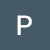Getting started with Angular Framework

In this article I would like to introduce Angular framework to beginners who are just getting started. This article will serve only as an introduction and further explanations and concepts of Angular will be publlished in my next few articles.

Angular is a framework for building mobile and web based…

Fundamentals of AWS Networking

Imagine you are the owner of a coffee shop. You have a cashier who takes orders from customers and a barista who prepares the coffee. Now, what if there are a few customers who want to give orders directly to the barista? It disturbs the barista who focuses on brewing…

Topological Sorting for Interviews

Topological sorting is the ordering of vertices in a graph such that for a directed edge (u,v) (from vertex “u” to vertex “v”), the ordering of u is lower than that of v.

It is labelling of vertices of graph ‘G’ such that if F(v) is the ordering of vertex…

Foundations of data systems — Part 1- A summary

This is my summary of the first chapter in the book, “Designing Data intensive Applications”

It is estimated that roughly 2.5 quintillion bytes of data is created every day. With the increase in IOT and sensors, from 2020 it is ensured that the global supply of data will be doubled…

Quick Select Algorithm

Quick Select is a variation of the quicksort algorithm. It is an optimized way to find the kth smallest/largest element in an unsorted array.

Algorithm:

• The partition part of the algorithm is same as that of quick sort.
• After the partition function arranges the elements in list according to the pivot…

Binary Search Problem : Search a 2D Matrix II [Leetcode 240]

This problem is similar to “Search a 2D matrix”, the solution to which I have written in my previous article. If you have read the previous article, read the problem description and continue to the efficient solution.

Problem Description:

Write an efficient algorithm that searches for a target value in an m…

Binary Search Problems- Search a 2D Matrix [Leetcode — 74]

Problem Description

Write an efficient algorithm that searches for a value in an m x n matrix. This matrix has the following properties:

• Integers in each row are sorted from left to right.
• The first integer of each row is greater than the last integer of the previous row.

Longest Consecutive Sequence — Leetcode 128 [Medium]

Given an unsorted array of integers nums, return the length of the longest consecutive elements sequence.

You must write an algorithm that runs in O(n) time.

Naive Solution:

A naive solution is sorting the given array and then do a linear traversal to find the longest consecutive length. It is an easy…

Triangle- Leetcode 120 [Medium]

https://leetcode.com/problems/triangle/

Given a triangle array, return the minimum path sum from top to bottom.

For each step, you may move to an adjacent number of the row below. More formally, if you are on index i on the current row, you may move to either index i or index i + 1 on the next row.

Solution

If you are at an index “i” in a row, you can move to index “i” or index “i+1” in the next row. So, the minimum value for an index “i” in a row can be obtained by considering index “i-1” or index “i” from the previous row. So, the original triangle matrix is modified top down considering the minimum values from the previous row. Finally, we return the minimum value from the last row.

LeetCode : Populating Next Right Pointers in Each Node I and II — [Medium]

Problem Description:

You are given a perfect binary tree where all leaves are on the same level, and every parent has two children. The binary tree has the following definition:

struct Node {
int val;
Node *left;
Node *right;
Node *next;
}

Populate each next pointer to point to its…Pruthvik Reddy

Looking for opportunities in software development. Interested in Blockchain, distributed computing and back-end development.